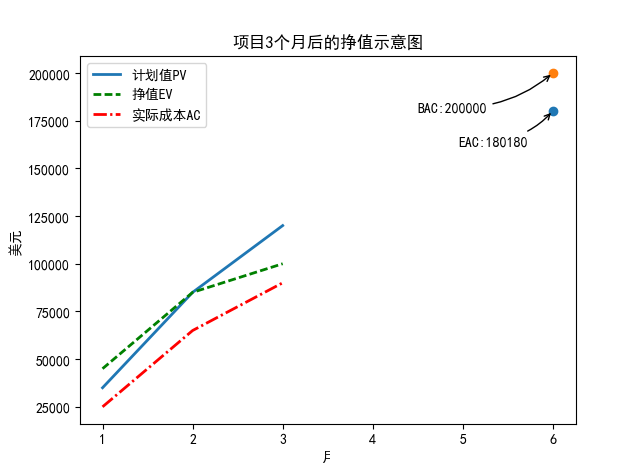• from urllib.request import urlopen...# 折线图 plt.plot(range(len(list_add_name)),list_add_num,marker = 'o',color='coral') # x，y轴的说明 plt.xlabel('国家',font1) plt.ylabel('数量',font1) plt.show()
from lxml.html import parse
from urllib.request import urlopen
import pandas as pd
import numpy as np
import matplotlib.pyplot as plt
from pylab import *
import csv
import re
t_data = pd.read_csv('D:\\kaola\\deal_with_data\\t_data.csv',encoding='GB18030')
# 分组
gp = t_data.groupby(by=['address'])
# 将得到的结果转化为DataFrame格式
data = gp.size()
data = data.reset_index(name='items')
# 按items升序排序
data = data.sort('items')
#重新设置索引
data = data.reset_index(drop=True)
# 定义两个列表
list_add_num = []
list_add_name = []
# 将产地名称加入list_add_name列表中
for i in data['address']:
print(i)
list_add_name.append(i)
# 将总数加入list_add_num列表中
for i in data['items']:
print(i)
list_add_num.append(i)

import matplotlib

#指定默认字体
matplotlib.rcParams['font.sans-serif'] = ['SimHei']
matplotlib.rcParams['font.family']='sans-serif'
# 设置画布大小
fig = plt.figure(figsize = (20,20))

font1 = {'family' : 'sans-serif',
'weight' : 'normal',
'size'   : 16,
}

#fig.suptitle('产地在各个国家所在的数量', fontsize = 24, fontweight = 'bold')
# 绘制图形
plt.bar(range(len(list_add_num)),list_add_num,align = 'center',color='red',alpha = 0.8)
# 标题
plt.title('产地在各个国家所在的数量')
# x轴设置
plt.xticks(np.arange(18),list_add_name)
#plt.xticks(x,list_add_name)
# y轴设置
#plt.ylim([0,500])
#plt.xlim([0,60])
# 设置条形图所代表的数量
for x,y in enumerate(list_add_num):
plt.text(x,y+20,'%s'%round(y,1),ha='center')
# 折线图
plt.plot(range(len(list_add_name)),list_add_num,marker = 'o',color='coral')
# x，y轴的说明
plt.xlabel('国家',font1)
plt.ylabel('数量',font1)
plt.show()
展开全文• python折线图方法前PPT要用到折线图，嫌弃EXCEL自带的看上去不好看，就用python写了一个画折线图的程序。import matplotlib.pyplot as pltx=[1,2,3,4,5,6]y1=[35000,85000,120000]y2=[45000,85000,100000]y3=...
python画折线图方法前做PPT要用到折线图，嫌弃EXCEL自带的看上去不好看，就用python写了一个画折线图的程序。import matplotlib.pyplot as pltx=[1,2,3,4,5,6]y1=[35000,85000,120000]y2=[45000,85000,100000]y3=[25000,65000,90000]point1=180180point2=200000plt.rcParams['font.sans-serif'] = ['SimHei'] #用来正常显示中文标签plt.rcParams['axes.unicode_minus'] = False #用来正常显示负号plt.scatter(x,point1,marker='o')plt.scatter(x,point2,marker='o')plt.plot(x[:3],y1,label='计划值PV',linewidth = 2)plt.plot(x[:3],y2,'g--',label='挣值EV',linewidth = 2)plt.plot(x[:3],y3,'r-.',label='实际成本AC',linewidth = 2)plt.ylabel('美元')plt.xlabel('月')plt.annotate('EAC:%d'%point1, xy = (6, point1), xytext=(5.5*0.9, point1*0.9),arrowprops=dict(arrow, connection)) #添加注释，即85%处的标记。这里包括了指定箭头样式。plt.annotate('BAC:%d'%point2, xy = (6, point2), xytext=(5*0.9, point2*0.9),arrowprops=dict(arrow, connection)) #添加注释，即85%处的标记。这里包括了指定箭头样式plt.title('项目3个月后的挣值示意图')plt.legend()plt.show()结果图：以上就是本文的全部内容，希望对大家的学习有所帮助，也希望大家多多支持码农之家。利用python画出折线图本文实例为大家分享了python画折线图的具体代码，供大家参考，具体内容如下# encoding=utf-8import matplotlib.pyplot as pltfrom pylab import *                 #支持中文mpl.rcParams['font.sans-serif'] = ['SimHei']names = ['5', '10', '15', '20', '25']x = range(len(names))y = [0.855, 0.84, 0.835, 0.815, 0.81]y1=[0.86,0.85,0.853,0.849,0.83]#plt.plot(x, y, 'ro-')#plt.plot(x, y1, 'bo-')#pl.xlim(-1, 11) # 限定横轴的范围#pl.ylim(-1, 110) # 限定纵轴的范围plt.plot(x, y, marker='o', mec='r', mfc='w',label=u'y=x^2曲线图')plt.plot(x, y1, marker='*', ms=10,label=u'y=x^3曲线图')plt.legend() # 让图例生效plt.xticks(x, names, rotation=45)plt.margins(0)plt.subplots_adjust(bottom=0.15)plt.xlabel(u"time(s)邻居") #X轴标签plt.ylabel("RMSE") #Y轴标签plt.title("A simple plot") #标题plt.show()效果图：以上就是本文的全部内容，希望对大家的学习有所帮助，也希望大家多多支持码农之家。以上就是本次给大家分享的关于java的全部知识点内容总结，大家还可以在下方相关文章里找到相关文章进一步学习，感谢大家的阅读和支持。
展开全文• 网上发现一个讲Python做柱状图和折线图的小视频，我觉得讲的超基础，很适合小白，特来分享给大家~如果你也想学习数据分析，跟我一起看看下方视频，听知名技术专家李刚老师对Python可视化模块详细解析，...
Python爬虫太火了，没写过爬虫，都不敢说自己学过Python？！可是刚一开始学我就遇到了难题----数据分析！听起来很高大上，有没有？想要做爬虫，就得先学会使用数据分析工具，制作图表这是最基本的。网上发现一个讲Python做柱状图和折线图的小视频，我觉得讲的超基础，很适合小白，特来分享给大家~如果你也想学习数据分析，跟我一起看看下方视频，听知名技术专家李刚老师对Python可视化模块详细解析，Python 数据分析李刚老师出版的《疯狂Java》系列图书曾得到市场的广泛认可，经过多次再版，已被多家高校选作教材。上方视频来自于李刚老师的在线视频课程《21天通关Python》第九章 数据分析鉴于大家都有学习Python的困惑，今天就给大家推荐一本巨有影响力的Python实战书，上线时间仅2个月，就超越了众多实力派，成京东和当当网上的长期畅销图书，并且收获了3.4W的五星好评。这本书可谓是笔者独家私藏图书之一了，对我学习Python有着莫大的帮助，在京东上也常常"断货"，这次拿出来给大家分享一下,希望能帮到大家。《21天通关Python》视频课程以畅销图书为教材，由图书作者李刚亲自操刀讲解；上手门槛低，可作为0基础掌握Python教材；书籍+线上复合型学习场景特别适合Python小白学习！笔者跟大家分享一个福利！下单时输入优惠码csdn66，立减20元，券后仅需99元！扫码入Python技术交流群，可免费听技术讲座+领学习资料+视频课免费看！展开全文• PPT要用到折线图，嫌弃EXCEL自带的看上去不好看，就用python写了一个画折线图的程序。 import matplotlib.pyplot as plt x=[1,2,3,4,5,6] y1=[35000,85000,120000] y2=[45000,85000,100000] y3=[25000,65000,...
• ## python画折线图

千次阅读 2017-11-05 21:41:18
之前PPT要用到折线图，嫌弃EXCEL自带的看上去不好看，就用python写了一个画折线图的程序。import matplotlib.pyplot as pltx=[1,2,3,4,5,6] y1=[35000,85000,120000] y2=[45000,85000,100000] y3=[25000,65000,...
之前做PPT要用到折线图，嫌弃EXCEL自带的看上去不好看，就用python写了一个画折线图的程序。

import matplotlib.pyplot as plt

x=[1,2,3,4,5,6]
y1=[35000,85000,120000]
y2=[45000,85000,100000]
y3=[25000,65000,90000]
point1=180180
point2=200000
plt.rcParams['font.sans-serif'] = ['SimHei'] #用来正常显示中文标签
plt.rcParams['axes.unicode_minus'] = False #用来正常显示负号

plt.scatter(x,point1,marker='o')
plt.scatter(x,point2,marker='o')
plt.plot(x[:3],y1,label='计划值PV',linewidth = 2)
plt.plot(x[:3],y2,'g--',label='挣值EV',linewidth = 2)
plt.plot(x[:3],y3,'r-.',label='实际成本AC',linewidth = 2)
plt.ylabel('美元')
plt.xlabel('月')
plt.annotate('EAC:%d'%point1, xy = (6, point1), xytext=(5.5*0.9, point1*0.9),
arrowprops=dict(arrowstyle="->", connectionstyle="arc3,rad=.2")) #添加注释，即85%处的标记。这里包括了指定箭头样式。
plt.annotate('BAC:%d'%point2, xy = (6, point2), xytext=(5*0.9, point2*0.9),
arrowprops=dict(arrowstyle="->", connectionstyle="arc3,rad=.2")) #添加注释，即85%处的标记。这里包括了指定箭头样式

plt.title('项目3个月后的挣值示意图')
plt.legend()
plt.show()

结果图：展开全文• matplotlib 是python最著名的绘图库，它提供了一整套和matlab相似的命令API，十分适合交互式地行制图。而且也可以方便地将它作为绘图控件，嵌入GUI应用程序中。 它的文档相当完备，并且Gallery页面中有上百幅缩略...
• from pylab import * mpl.rcParams['font.sans-serif'] = ['SimHei'] # 添加这条可以让图形显示中文 x_axis_data = [2000,2001,2002,2003,2004,2005,2006,2007,2008,2009,2010,2011,2012,2013,2014,2015] ...
• 折线图的绘制主要分为四步： 1）导入相关的库 2）图片输出的通用设置 3）设置数据及折现的属性 4）设置图的标题 案例： import matplotlib import numpy as np from matplotlib import pyplot as plt %...matplotlib
• 网上发现一个讲Python做柱状图和折线图的小视频，我觉得讲的超基础，很适合小白，特来分享给大家~ 如果你也想学习数据分析，跟我一起看看下方视频，听知名技术专家李刚老师对Python可视化模块详细...学习编程
•   Python中的折线图可用于分析类似温度，市场等数据的变化趋势等。本人的小练习是分析一段数据中各任务的运行时长，检测出异常数据（运行时间超出正常时间范围的任务）。自学可在网上下载一些数据作分析。 ...
• 我正在从Wind金融终端里获取数据并用pptx生成折线图最后自动生成ppt的程序，求教折线图的刻度表怎么设置？比如默认刻度表从0开始间距1000，我想设置成3000开始，间距500，另外X轴...
• 今年是很值得记录的一年，上半年在家大半年，由于自律运动锻炼体重下降到了史上最低值，自返校后，我就尽量每天晚上记录体重，年末，我就用体重值和自己的学的数据分析技能简单的了份总结。 一、数据解释 真正开始...数据分析 matplotlib
• ![图片说明](https://img-ask.csdn.net/upload/201803/26/1522023911_68604.png) python折线图，想要把每条线的标志部分移到外面的空白红色框中，该如何
• 在使用python的时候经常会出现各种数据，有的时候光看数字还感觉不出来...###折线图和柱状图#### import numpy as np import matplotlib.pyplot as plt # ========================================= x = np.arr...
• 然后折线图，并将折线图插入到原本的excel中。 我在网上百度了很久，看到有用xlsxwriter的worksheet.insert_chart方法，但是这个方法好像是将原始数据复制到里另一个excel中并在新生成的...
• Python数据分析之matplotlib折线图1.需求2.需求分析3.代码示例4.总结和思考 1.需求 需求假设大家在30岁,根据实际情况,统计出你和同桌从11岁倒30岁成的男女朋友数量,绘出折线图分析自己和同桌的谈恋爱走势 ‘’’ ...数据分析 数据可视化 matplotlib
• 在matplotlib中使用plot()函数可以做出简单折线图，预期效果如下： 代码如下： # 导入pyplot模块 import matplotlib.pyplot as plt # 输入横纵坐标数据 months = [1,2,3,4,5,6] people = [50,60,45,30,70,65] plt...
• 网上发现一个讲Python做柱状图和折线图的小视频，我觉得讲的超基础，很适合小白，特来分享给大家~ 如果你也想学习数据分析，跟我一起看看下方视频，听知名技术专家李刚老师对Python叠加柱状图和...学习编程
• 应该是html一类的，但还没学过这个，希望各位大佬给出简单易懂的解答。</p>
• python使用matplotlib绘制折线图教程 https://www.cnblogs.com/onemorepoint/p/7482644.html 没找到上传excel附件的位置，把图片贴出来对数据一个说明： 第1行为X周，是时间单位，由于是相对时间，所以直接用数字...excel pycharm
• 网上发现一个讲Python做柱状图和折线图的小视频，我觉得讲的超基础，很适合小白，特来分享给大家~ 如果你也想学习数据分析，跟我一起看看下方视频，听知名技术专家李刚老师对Python可视化模块详...学习编程
• 最近在工作中接触到了数据分析，其中需要绘制折线图，在经过一番查找和改善之后，最终写了一个自用的函数，在此特保留，以防丢失 目录 Code 使用方法 示例图 写在后面 Code # 绘制图表 def plt_show(x: dict,...可视化 数据可视化 matplotlib
• #用python绘制工件的图像import pandas as pdimport numpy as npimport matplotlib.pyplot as pltfrom mpl_toolkits.mplot3d import Axes3Dcsv_data = np.loadtxt(open('/home/yifei/Proj/PythonProj/getboundary/...
• python的matplotlib库绘制条形图、散点图、饼图、折线图 当我们学会了爬虫，抓取到了一些数据，接下来就是数据分析了。本文章介绍绘制图形的基本代码。 打开cmd用pip 安装，若输入pip install matplotlib，文件...数据可视化
• 写在前面：为啥不用excel绘制这些...所以，要学术的朋友，对R，Python可视化绘图感兴趣的朋友，不妨下点功夫将这些技术学到手。 我之所以，将自己花费4个晚上，8个小时整理出来的文档分享出来，无非是为了减少大...
• 使用python读取excel中的数据，并绘制折线图 实验的时候采集到一些数据，从文本拷贝到excel，然后从十六进制转换成十进制。图表时分析数据的有利工具，使用python绘制出的图标简明美观。所以我这次尝试一下。...excel
• 以下展示一些用 matplotlib 画条形图、折线图、饼图以及散点图的示例，其中类似于图例、坐标轴名称，标题等的显示方法是一样的，不另介绍。 0、引入模块 import random import matplotlib.pyplot as plt import ...
• python读取Excel数据，并绘制折线图数据分析的时候，用到了对Excel中的数据进行显示处理，能更直观的了解数据，总结数据中蕴含的规律和信息。 import matplotlib.pyplot as plt import xlrd from xlrd import ...数据分析 数据可视化...

# python做折线图python 订阅